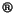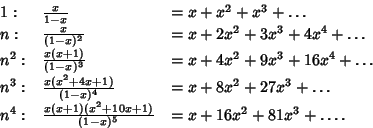## Generating Functionwhose Coefficients give the Sequence,, .... The Mathematica(Wolfram Research, Champaign, IL) function DiscreteMathRSolvePowerSum gives the generating function of a given expression, and ExponentialPowerSum gives the exponential generating function.

Generating functions for the first few powers are